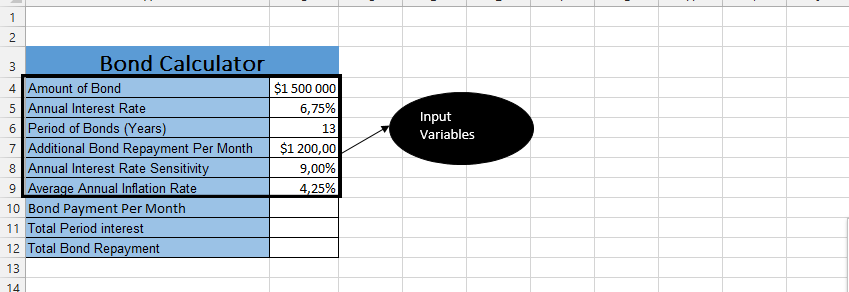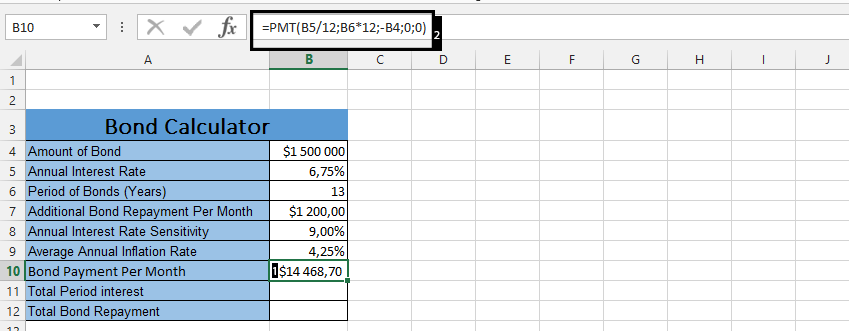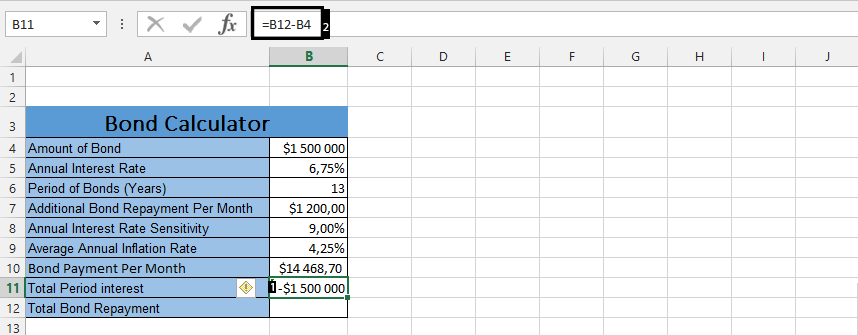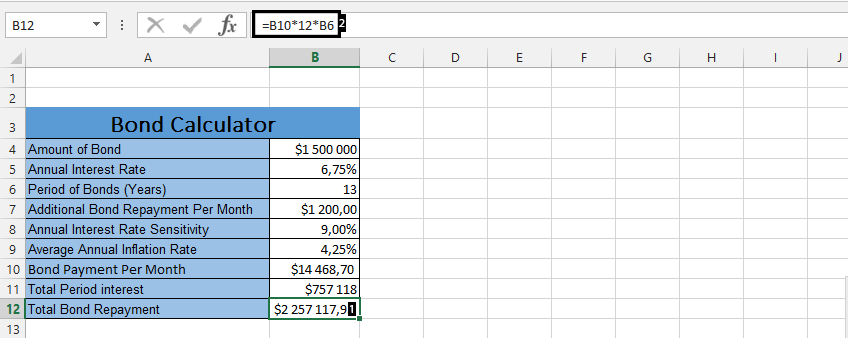#### How to Create Bond Repayment Calculator

In this Excel tutorial, you will learn how to prepare bond repayment calculator in Excel.

## How to create bond calculator?

A bond repayment calculator in Excel can help you determine the payment schedule and total interest paid on a bond over time. Here’s how you can create one:

To create a bond repayment calculator in Excel, you will need the following data:

• Bond amount
• Annual interest rate
• Number of yearsPut in the values of your choice. They act as components in the bond calculation.

## Bond repayment formula

Calculate the payment amount. Use the “PMT” function in Excel to calculate the payment amount. The PMT function requires the rate, number of payments, and present value as inputs.

Type =PMT(B5/12;B6*12;-B4;0;0) next to Bond Payment Per Month.Note: The =PMT(annual interest rate/12(number of months in a year);Period of Bonds*12(also number of months in a year). Place a minus symbol before the bond amount. This minus symbol will make it possible to get an accurate result.

Click beside total period interest and type =B12-B4.Click beside total bond repayment (1) and type =B10*12*B6.These steps should help you create a bond repayment calculator in Excel. This can be a useful tool for investors and analysts who want to understand the payment schedule and total interest paid on a bond over time. Keep in mind that you may need to make adjustments to the calculator depending on the specific bond and payment schedule.

## Using the Bond Repayment Calculator to Analyze Different Scenarios

Once you have created your bond repayment calculator, you can use it to analyze different scenarios. For example, you can use it to see how the monthly payment amount, total interest paid, and total bond repayment amount change when you:

Change the interest rate:

`=PMT(new interest rate / B4, B3 * B4, -B1, 0, 0)`

Change the term of the bond:

`=PMT(B2 / B4, new term * B4, -B1, 0, 0)`

Change the number of payments per year:

`=PMT(B2 / (new number of payments per year), B3 * (new number of payments per year), -B1, 0, 0)`

Change the face value of the bond:

`=PMT(B2 / B4, B3 * B4, -new face value, 0, 0)`

You can also use the calculator to compare different bonds. For example, you can compare two bonds that have different interest rates, terms, or payment frequencies.

By using your bond repayment calculator, you can gain a better understanding of how different factors affect the cost of a bond. This information can be helpful when making investment decisions.